# Sketching Translations and Reflections

## Sketching translations

Now that we have looked at the different types of graphs that you need to sketch, we need to look at how we translate graphs. Remember that each graph is a function of x which can be written as y = f(x). For this example f(x)= x2, which is the graph below: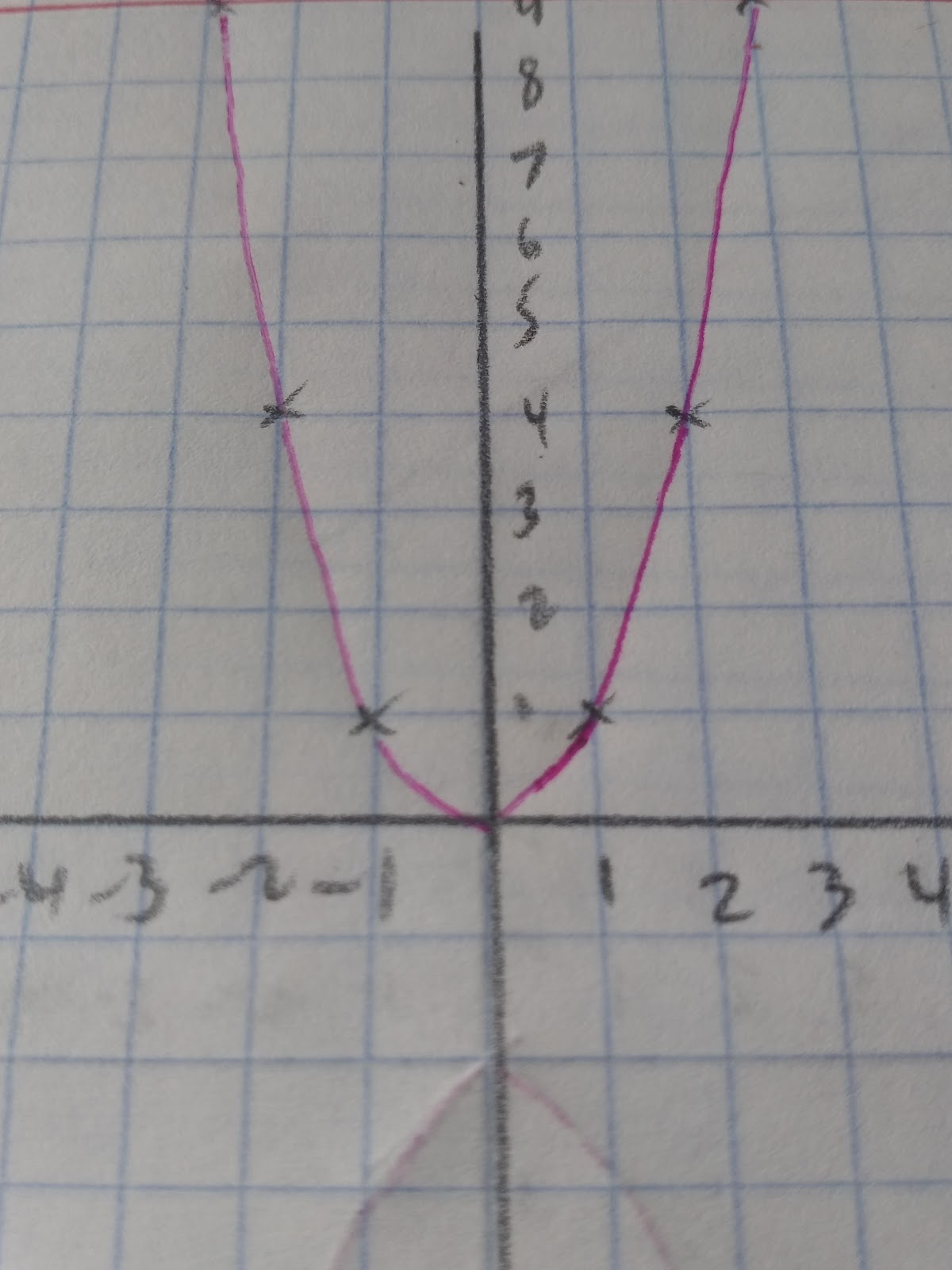When f(x) is translated by +k, to be f(x) +k, the equation becomes y=f(x) +kEg. When k=2 y=f(x)+2. The graph has moved up two units on the y axis so now crosses the y axis at (0,2). y=f(x) -2 would move the graph down 2 units on the y axis.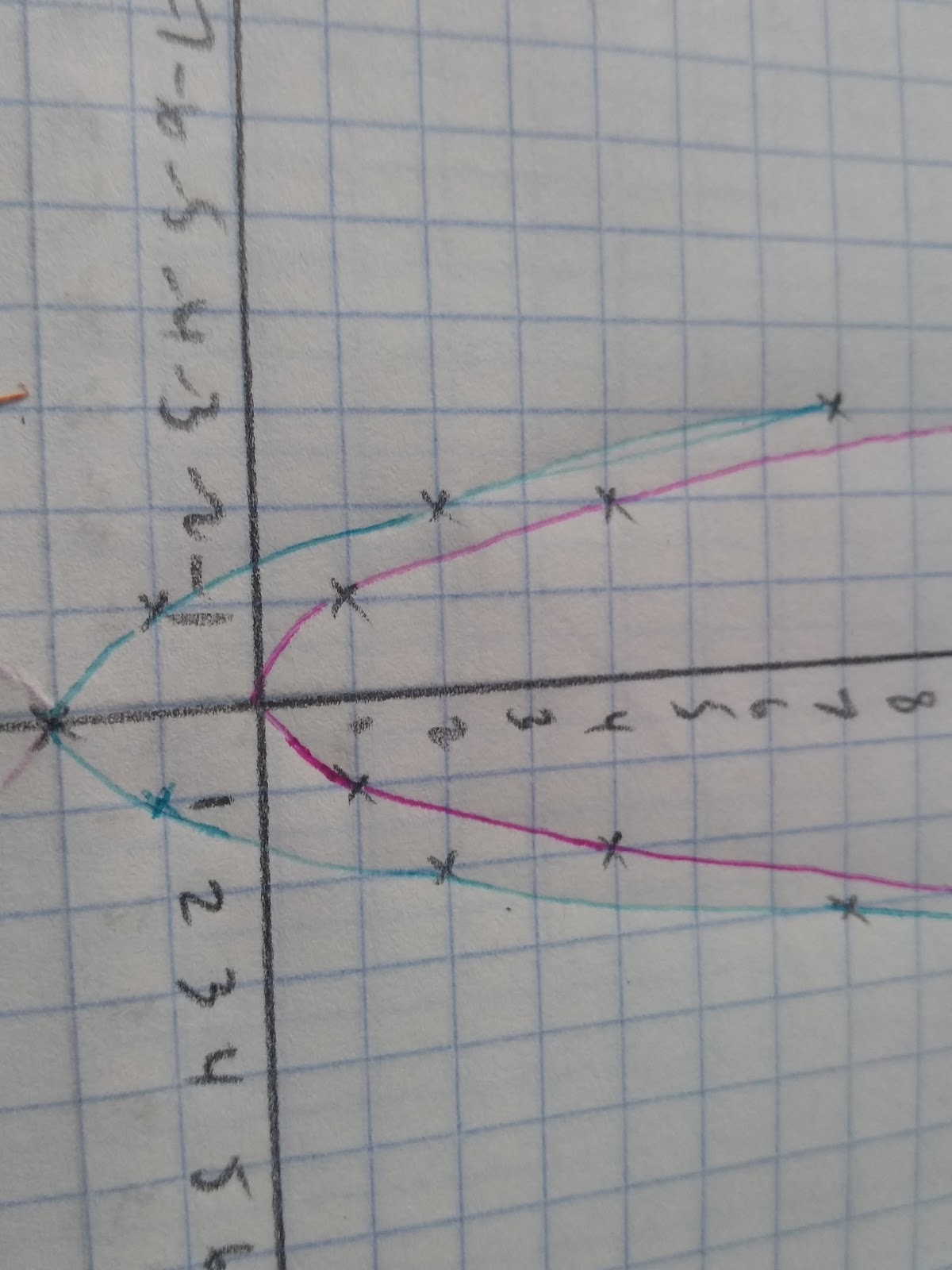y=f(x+k) This will shift the graph ‘k’ amounts along the x axis. When we +k the graph moves k amount to the left. f(x +2) moves the graph two places to the left.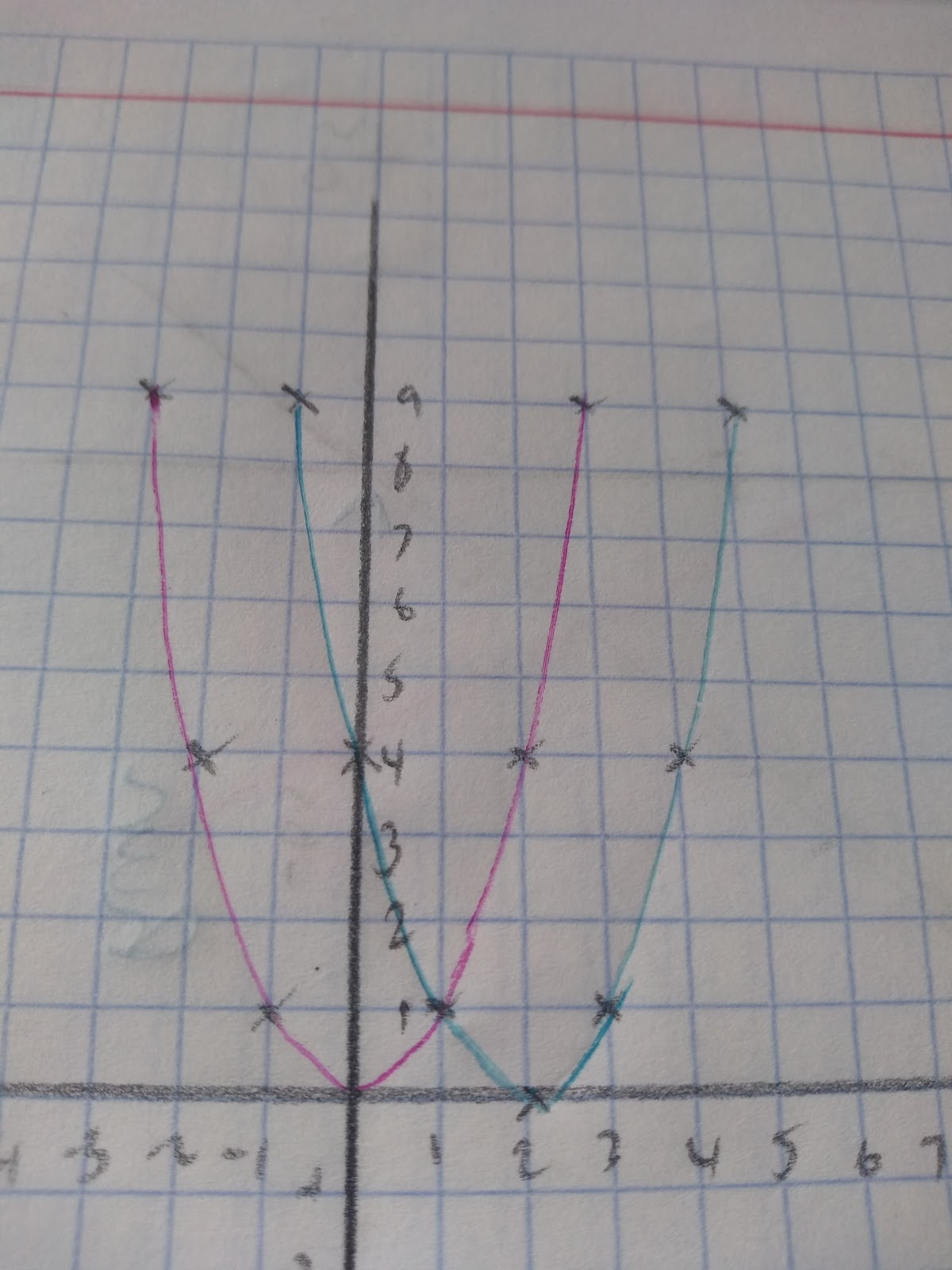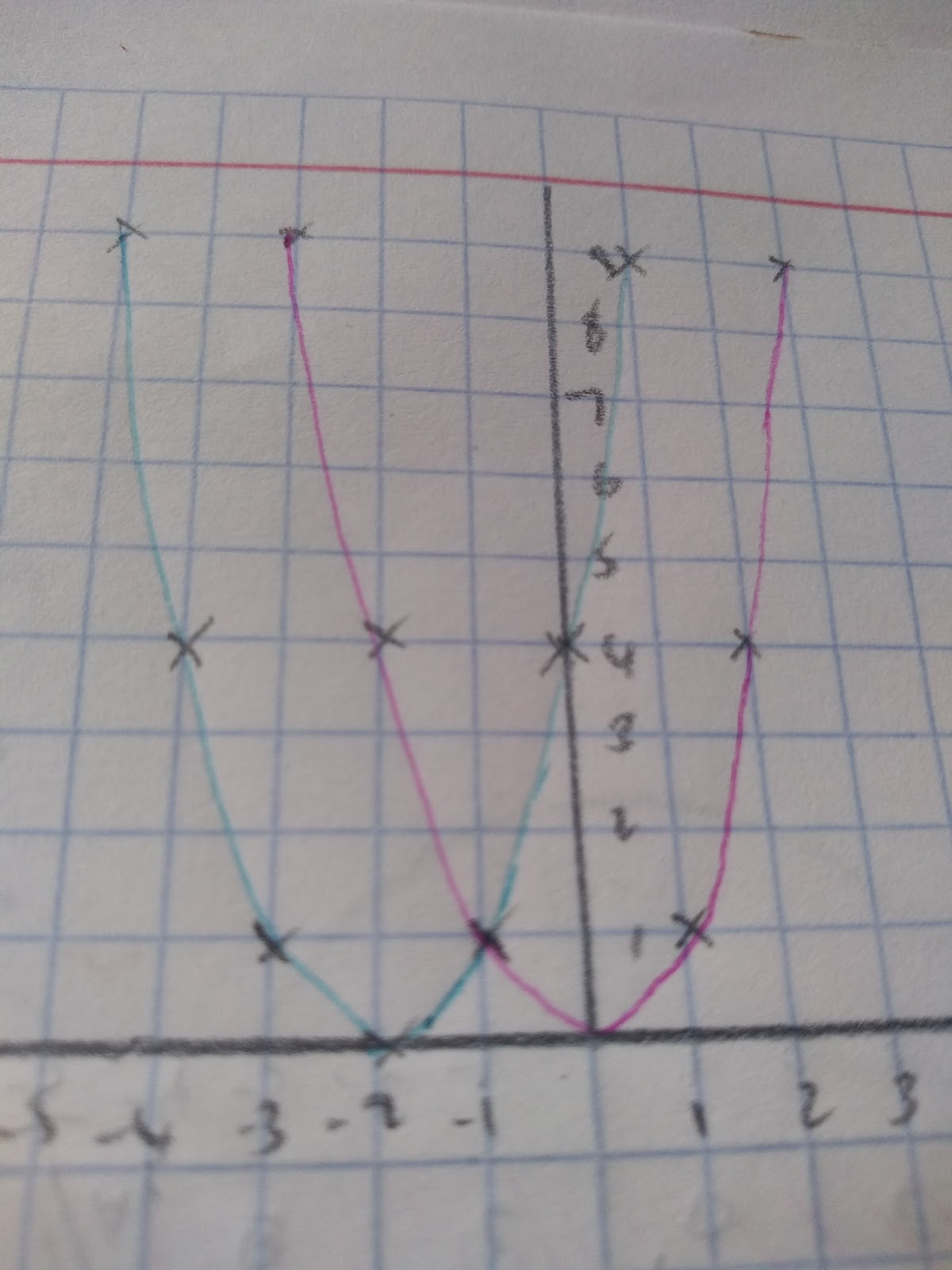y=f(x-2) moved the graph two places to the right:

Up: y=f(x)+k

Down: y=f(x)-k

Left: y=f(x+k)

Right: y=f(x-k)

## Sketching reflections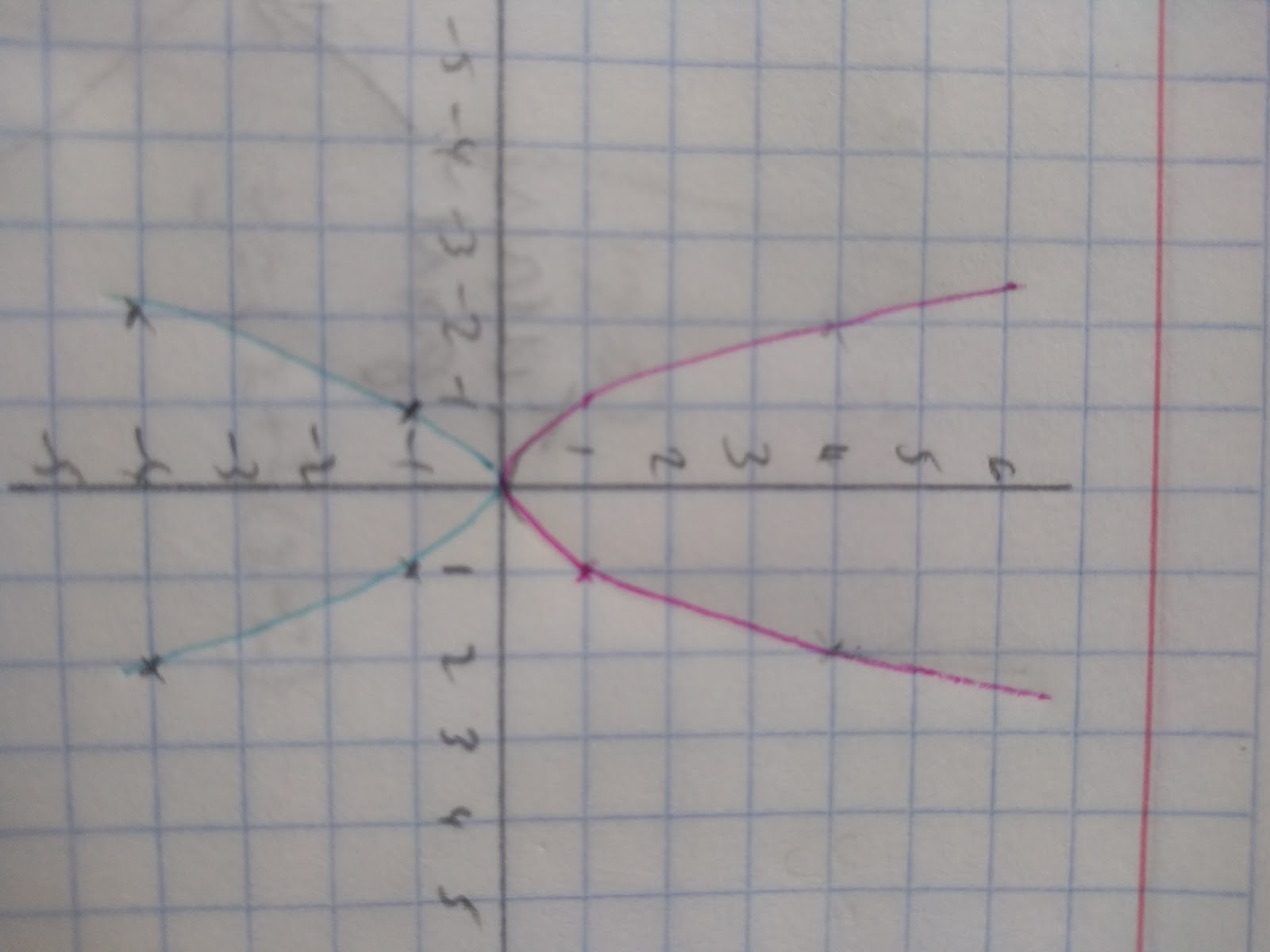y=-f(x) reflects the graph around the x axis: The reflection in the y axis is f(-x) To show this I am going to use the graph f(x)=x3+ 3 (as y=x2 will look the same as it’s a symmetrical through the y axis).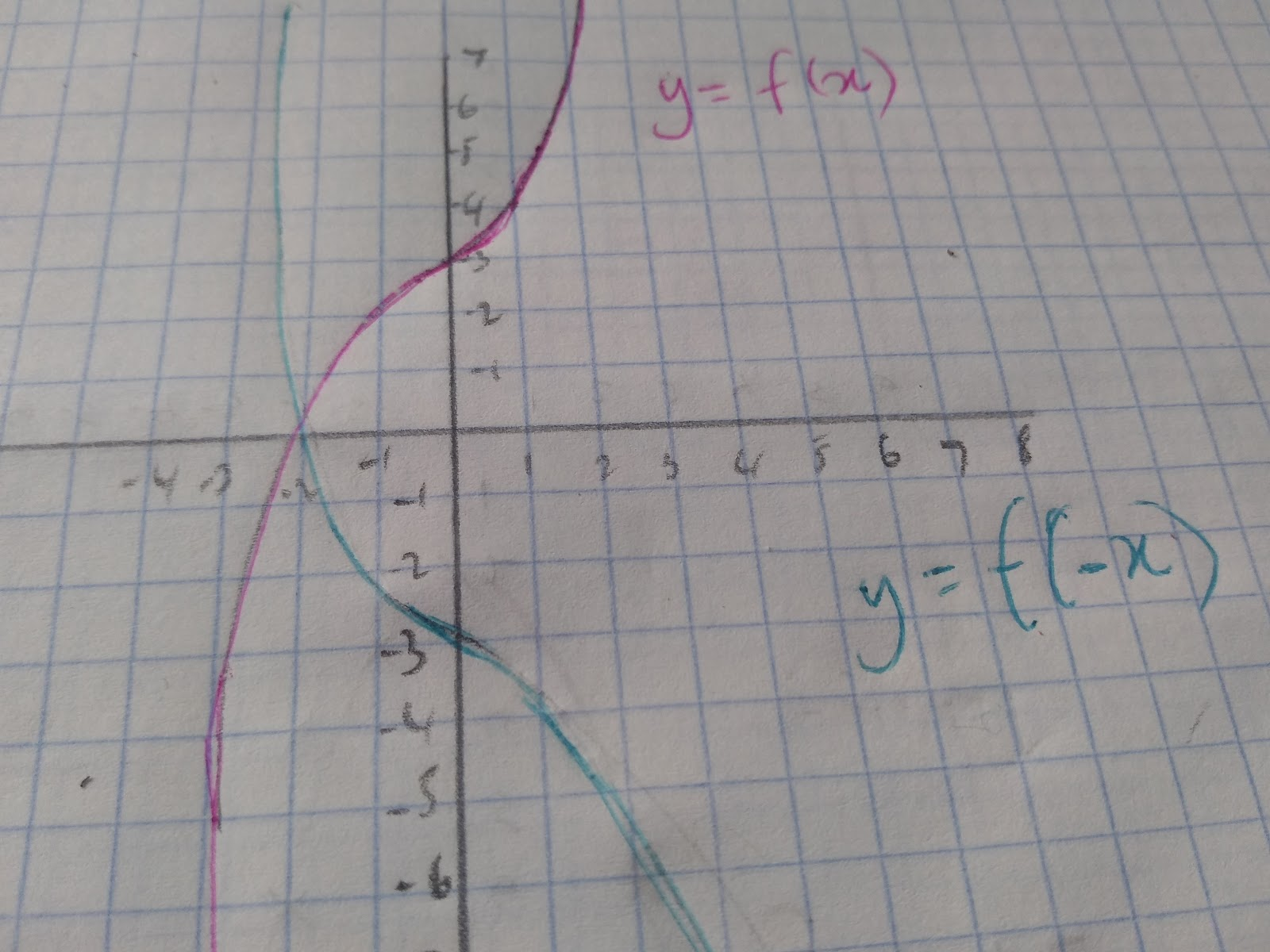When it comes to reflections you also need to know how to sketch inverse operations.

Key Fact: Inverse operations are always reflected in the line y=x. For example, the inverse of a logarithm is an exponential function.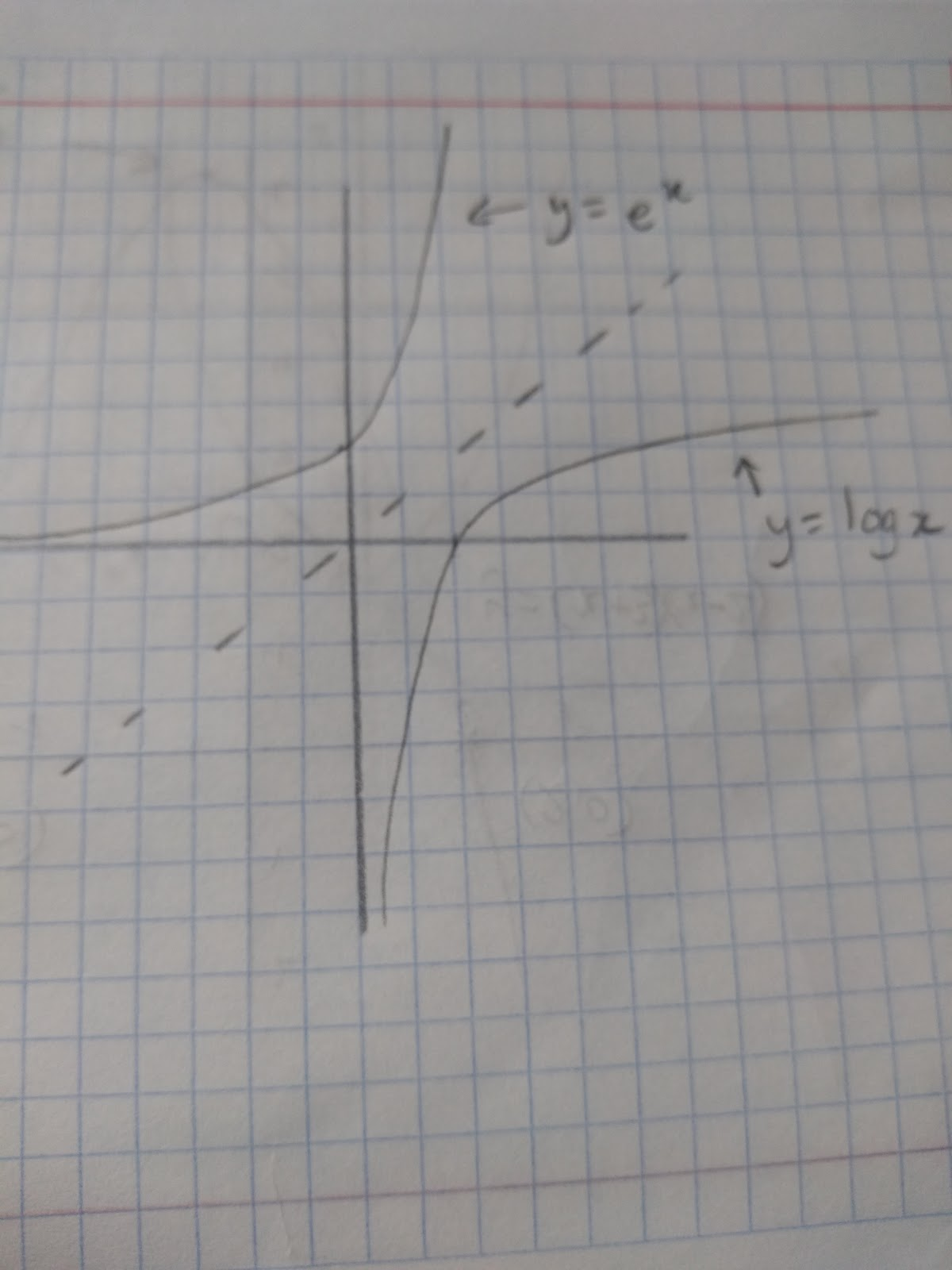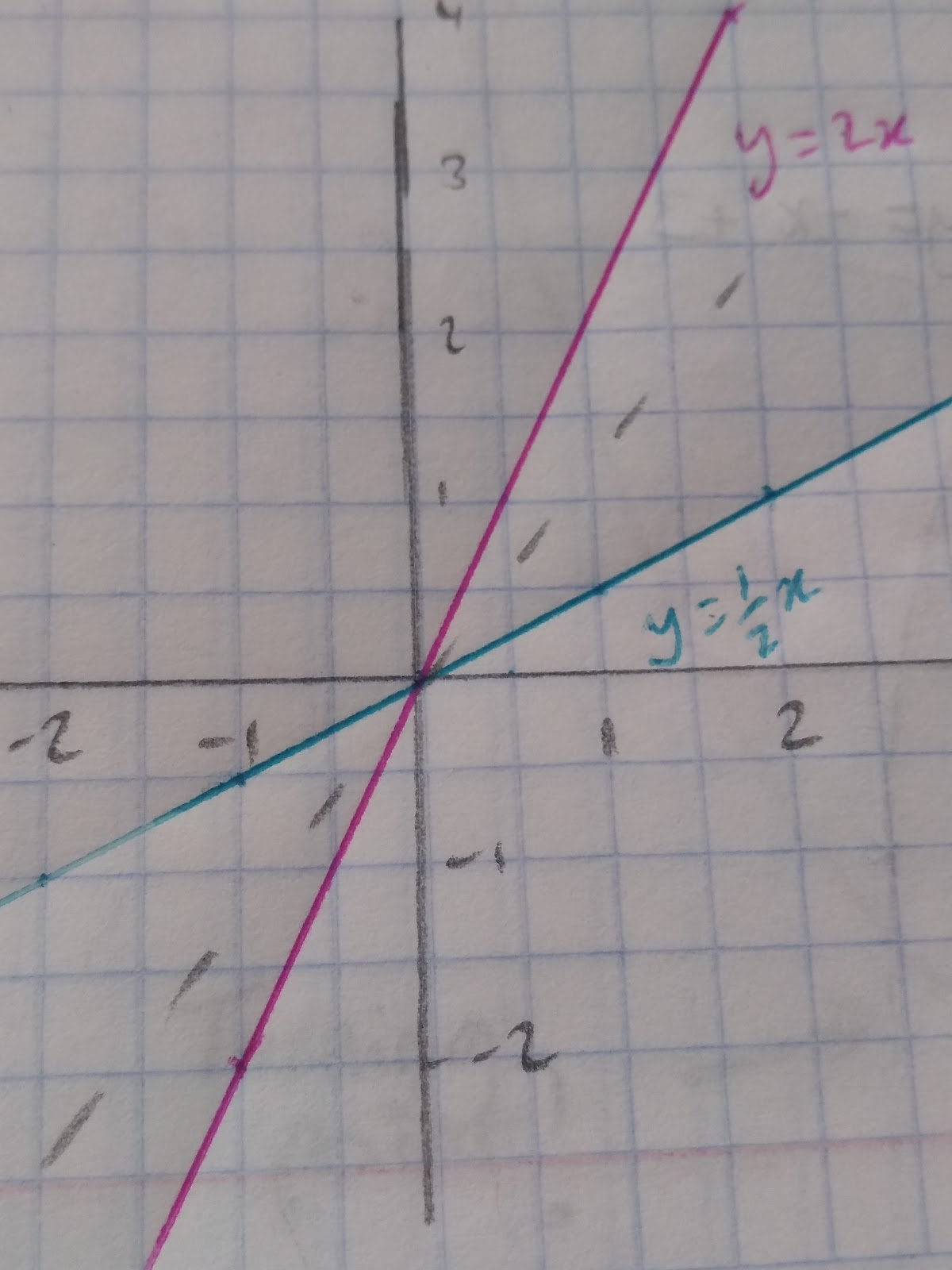As you can see any function’s inverse is symmetrical through the mirror line y=x. f(x) is always a reflection in y=x of f-1(x)

Sketching Enlargements

y=k(fx) is an enlargement of scale factor k in the y direction. Here we show an enlargement of a sine graph.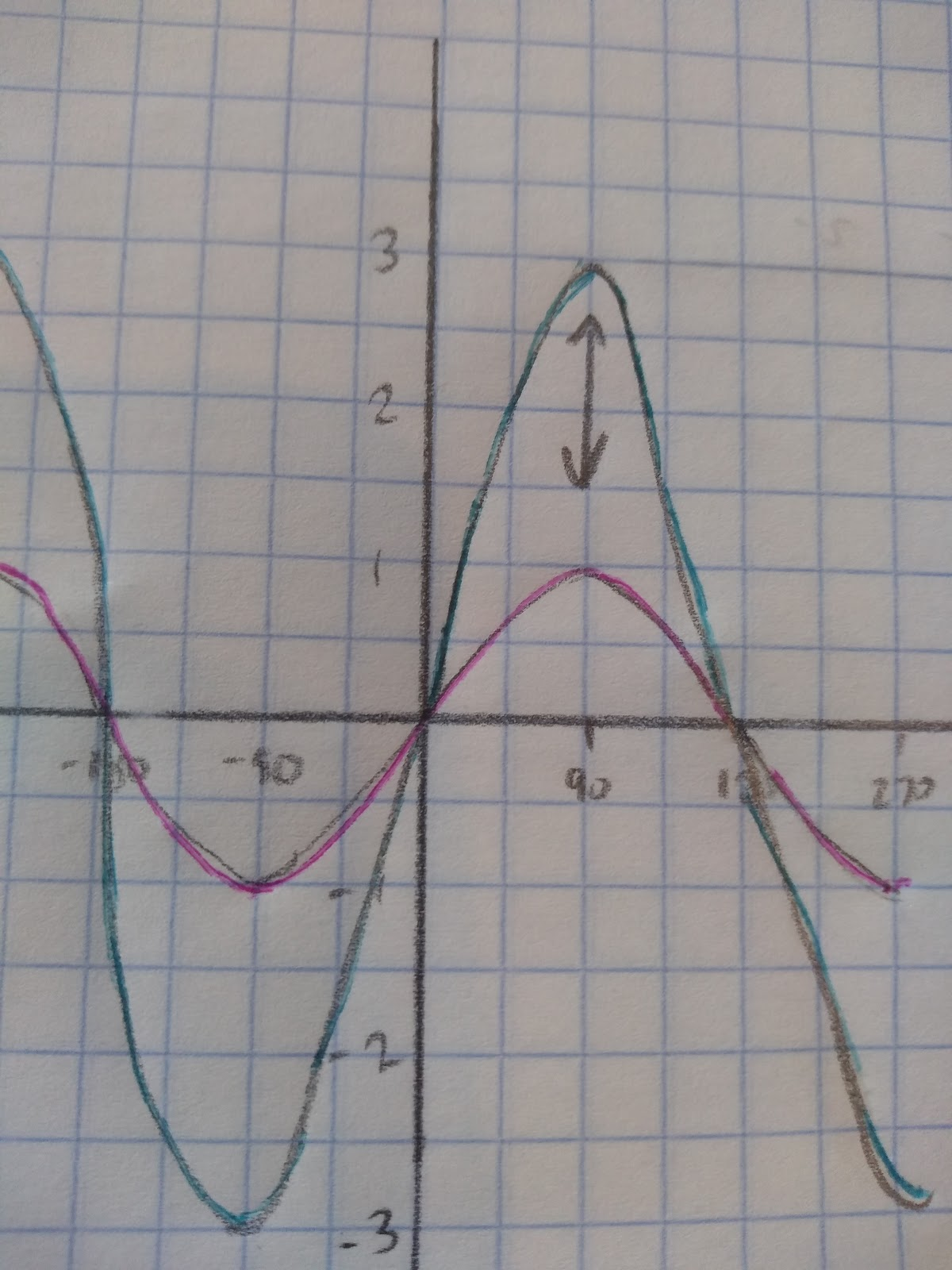y=sinx and y=3sinx. You can see the stretch is in the y axis.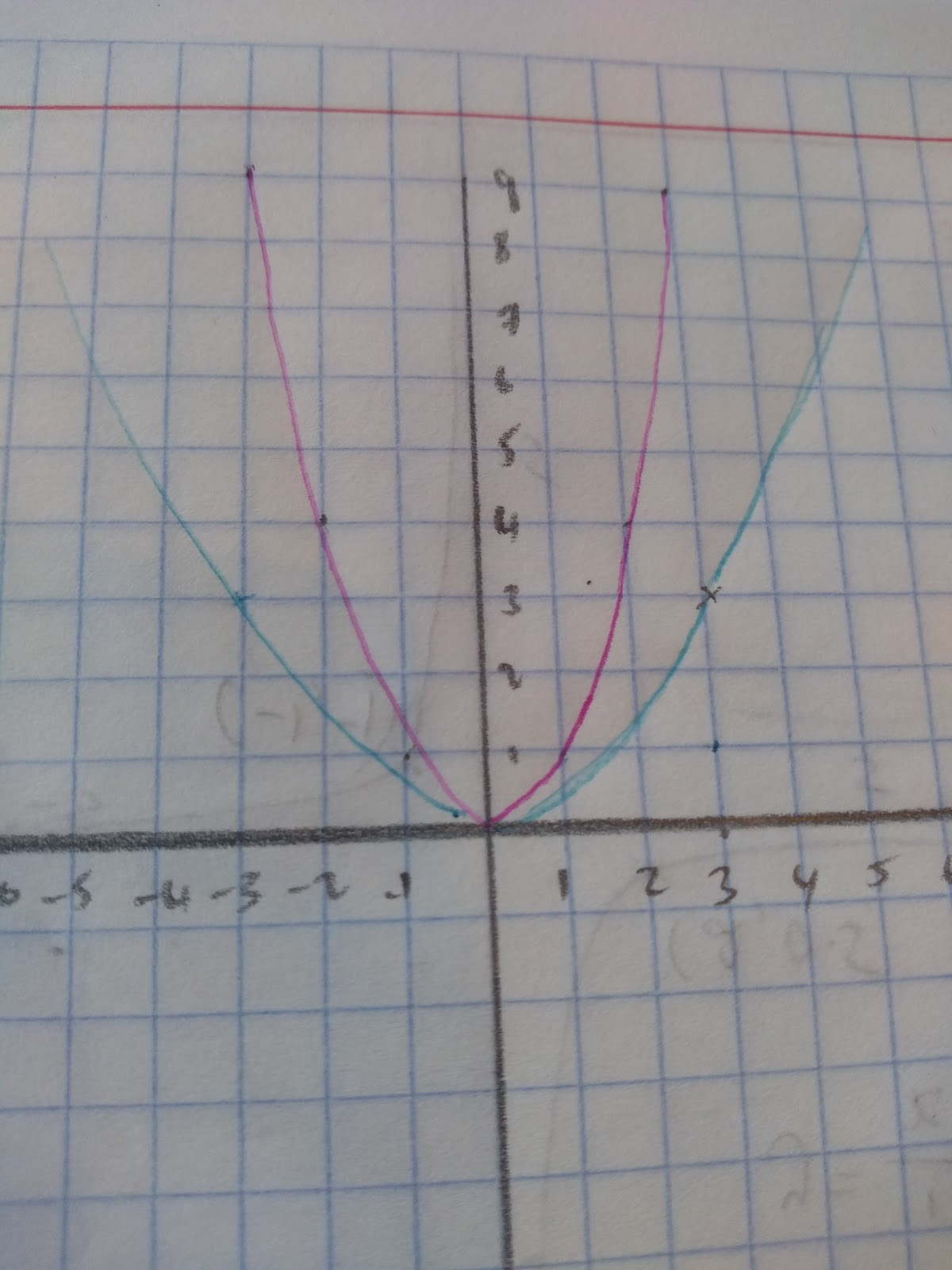y=x2 to y=1/3x2. y=f(kx) is an enlargement of scale factor 1 in the x direction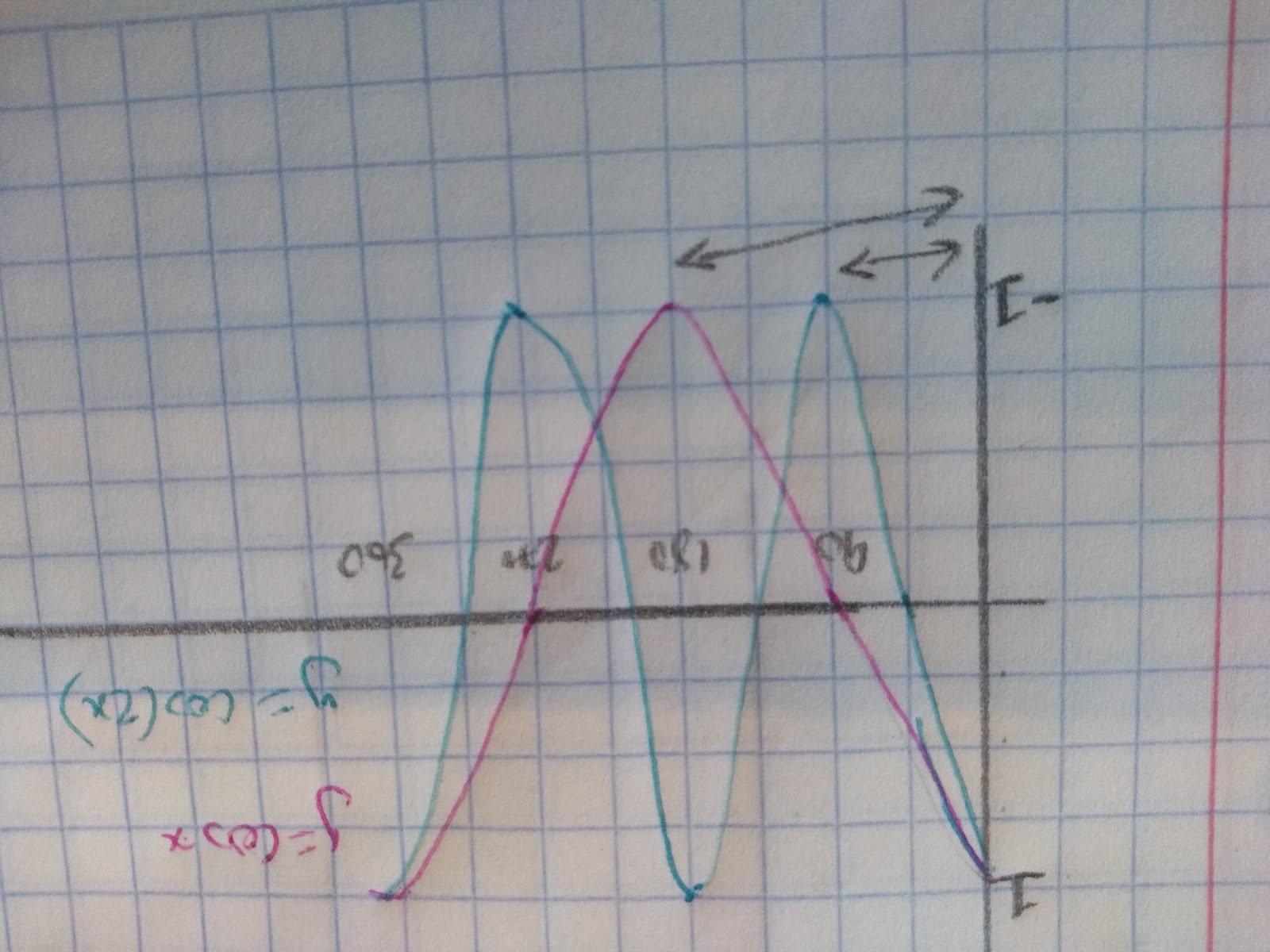The waves double because in this example we’ve gone from y=cosx to y=cos(2x), which squishes the graph up 2 more times!